﻿

Mathematical Constants - A Few Notes on Computation and Verification

By Alexander J. Yee

This page presents a few details on how each of the constants were computed and verified.

One thing to remember is that the program used to compute these constants uses a binary representation. Therefore, obtaining the decimal digits would require a base conversion from binary to decimal.

The Square Root Constants: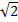,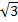, and Golden Ratio φ

All three were computed using first order Newton's Method.andwere verified by simply squaring them. The Golden Ratio was verified by plugging it back into its quadratic equation.

The decimal digits ofand Golden Ratio φ (and thus, the base conversions required to obtain them) were verified using outside sources. The decimal digits ofand the hexadecimal digits of all three were not verified.

My Thoughts

All three of these constants are by far the easiest implement and fastest to run.

An efficient implementation can compute n digits (of any of the three constants) faster than an n x n digit multiplication.

(From a practical perspective, an n-digit base conversion would take much longer to perform.)

Euler's Constant: e

e was computed using its Taylor expansion.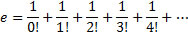This series was summed up using Binary Splitting to obtain maximum efficiency.

The decimal digits were verified using outside sources.
Successful verification of the decimal digits automatically implies that both the computation and the base conversion were correct. The hexadecimal digits were not verified.

My Thoughts

e is arguably the easiest (and fastest) of all the binary splitting constants to implement.

The fact that the entire binary splitting routine for e can be done entirely using integer arithmetic by itself already merits this position. (among other things)

Anyone wishing to write a program to compute (the more popular) π, should program e first since it is much simpler and is a good "stepping stone" to the more difficult-to-implement constants. (like π)

π

π was computed using the Chudnovsky formula.This series was summed up using Binary Splitting to obtain maximum efficiency.

The decimal digits were verified using outside sources.
Successful verification of the decimal digits automatically implies that both the computation and the base conversion were correct. The hexadecimal digits were not verified.

My Thoughts

As the formula shows, π, as harmless as it may seem, is a different animal from e.

Unlike that of e, the fastest (known) formula for π is not as innocent looking and as a result, practical implementation of π is much more difficult than e.

Here are some of the notable differences.

 1 First of all, the binary splitting process is much more complicated than that of e. There are more variables needed, more memory, more operations... etc. 2 Secondly, floating point arithmetic is needed for the binary splitting process for reasons described here. 3 And lastly, it is difficult to optimize the recursion. (which makes it difficult to write a π program that is as fast as some other top π programs.)

Overall, π is still an easy constant implement. But, because of it's popularity and the difficulty of optimizing it, it is extremely difficult to make it as fast as fastest π programs available on the web.

The Logarithmic Constants:andBoth were computed using machin-like formula.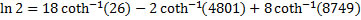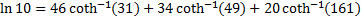Each of the Inverse Hyperbolic Cotangents were computed using taylor series along with Binary Splitting.

The decimal digits of both computations were verified using outside sources.
Successful verification of the decimal digits automatically implies that both the computations and both the base conversions were correct. The hexadecimal digits of neither constant were verified.

My Thoughts

The binary splitting routine for inverse cotangent is very similar to that of the Chudnovsky formula for π. So most of the difficulties also carry over.

As with π, logarithms of integers (assuming you know the formula for them) are relatively easy to implement.

However, even though the series for inverse cotangent is simpler than that of π, they converge slower.
This, combined with the fact that there are multiple sums, makes computing logarithms slower than computing π to the same precision.

Lemniscate and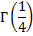A close observation of the Brent-Salamin AGM iteration for π reveals that it can be used to simultaneously compute π, Lemniscate, and.

Here is the well known Brent-Salamin AGM formula for π:By breaking up the formula, we obtain two constants, P and Q.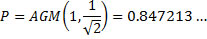Using P and Q, we can easily compute π, Lemniscate, andusing the following formulas.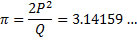π was verified by comparing with outside sources. Lemniscate andwere verified using the following identity: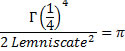It should be noted that this entire process only verifies the computation itself (not the decimal and hexadecimal digits).

My Thoughts

The AGM is quite possibly one of the most spectacular algorithms.

It's easy to implement, quadratically convergent, and can be used to compute a ton of constants. It can also be used to evaluate the natural logarithm at any point (even complex) in O(n log(n)2) time.

It doesn't stop there. Using Newton's Method to invert natural log, the exponential function can also be evaluated at any point in O(n log(n)2) time.

And it gets even better...

All trigonometric functions can be expressed in terms of the exponential function and all inverse trigonometric functions can be expressed in terms of the natural logarithm. (with the help of complex numbers)

Therefore, the AGM permits to compute ALL trigonometric functions and their inverses in O(n log(n)2) time.

Aside from trig functions, the AGM can also be used to compute complete elliptic integrals.

As far as constants are concerned, the AGM-based algorithms for π have the best known complexity of O(n log(n)2) whereas the series formulas are all O(n log(n)3).

However, because full precision is required for all iterations of the AGM, the big-O constant is typically huge - to the point where most of the series formulas are still faster well into the billions of digits.

Nevertheless, AGM has it's own benefits. (such as shorter code)

Catalan's Constant G

Catalan was computed using the following infinite series:The series was summed up using Binary Splitting to obtain maximum efficiency.

The natural log was computed using AGM.

The decimal digits were verified using outside sources.
Successful verification of the decimal digits automatically implies that both the computation and the base conversion were correct. The hexadecimal digits were not verified.

It should also be noted that Catalan's Constant, when compared to all the constants discussed above, is rather computationally intensive to compute.
Therefore, only 20 million digits have been provided.

My Thoughts

Don't let this rather simple formula fool you into thinking it's great. Because it isn't.

First of all, it is anything but fast. Its rate of convergence is only 2 bits/term. By comparison the Chudnovsky Formula for π gets roughly 43 bits/per term.

Therefore it is horrendously slow. There are faster known formulas Catalan's Constant (most notably the Zeilberger-type formula), but they still aren't much faster.

And secondly, from a practical perspective, the binary splitting routine for this series is more complicated than that of e, π, and the logs.

The more sophisticated binary splitting routing combined with the slow rate of convergence of the formula makes Catalan's Constant the slowest to compute of all the constants discussed so far.

Euler-Mascheroni Constant γ

Euler's Constant, γ, was computed using the Brent-Mcmillan formula (or rather an approximation).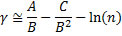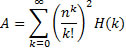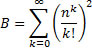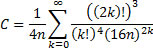Where n is the cutting parameter that determines the number of digits the approximation is correct to.

For a given n, the formula gives approximately 3.47435n correct decimal digits where:So using n = 225 the approximation is accurate to about 116,580,037 digits. (The actual value being 116,580,041 digits.)

For verification, n = 226 was used. (Note that powers of 2 were used in both computations since it permits to re-use the ln(2) code.)

Like Catalan's Constant, Euler's Constant is also extremely slow to compute.

My Thoughts

The Euler-Mascheroni Constant is not for the faint hearted. Just sheer complexity of the formula alone is enough to make Catalan's Constant seem like child's play.

Add to that the following difficulties that arise when attempting to implement the formula:

 1 Series C does NOT converge. One must be careful not to sum too many terms or it will blow up.This is a rather minor issue compared to the following two. 2 The rate of convergence of all three summations is irregular. (And series C doesn't even converge!) This has significant implications for optimization. Otherwise, it can mostly be ignored. 3 Series A is (in some sense) a double summation. The "standard" binary splitting approach does not work. This is a BIG problem and is what sets this constant apart from the rest of the other series-based constants.

These difficulties (mainly the last one), combined with the overall unpopularity of the constant is the reason why few people have given it much attention.
Resolving the last problem is essential to obtaining the quasi-linear run-time complexity that is required to efficiently compute this constant to millions of digits.
(Although there are other, but slower, formulas for γ that don't have a double series and therefore, do not have this issue.)
(hence, there are few programs on the web that can compute it and hence, why the world record was allowed to stand unbroken for 7 years)

Here's a table that compares the relative run-times for each of these constants at 1 million digits.

To put things in to perspective, the multiplication times have been included in the table.

 Constant BigNumber* BigNumber* Mathematica 5.2 Mathematica 6.0 n x n Multiplication 0.476 0.181 0.531 0.219 Base Conversion 6.918 3.701 ~5.5 ~30.855 0.389 0.734 0.3280.840 0.4 0.735 0.265 Golden Ratio φ 0.889 0.424 0.734 0.297 e 5.039 2.044 4.453 1.640 π 20.755 8.582 14.516 3.95438.049 15.723 52.093 13.62546.503 19.101 85.36 31.610 Lemniscate 65.906 22.736 - - Catalan G 500.288 189.608 366.281 82.000 Euler's Constant γ 313.006 158.847 1096.19 200.360

Note that none my implementations (with the exception of Euler's Constant γ) are optimized at all.

*BigNumber is the java library written entirely by me. The entire library was written in java with the exception of multiplication - which was written in C and linked using JNI.

The times in the first column correspond the same version of the library that was used to compute 116 million digits of Euler's Constant γ in December 2006.

The times in the second column were done using exactly the same java library, but with the native C multiplication switched out and replaced with the prototype FFT code of BigNumber 2.